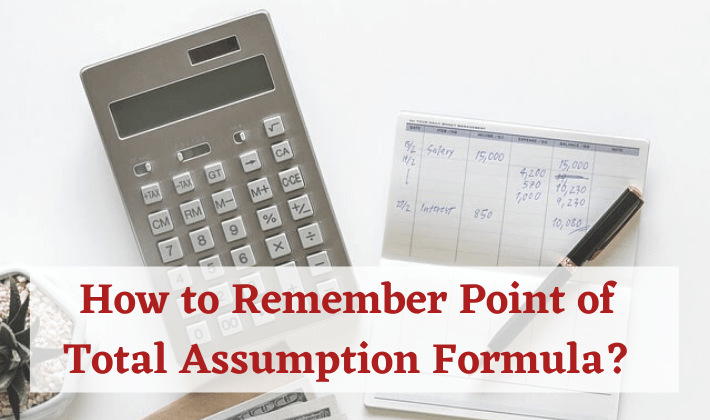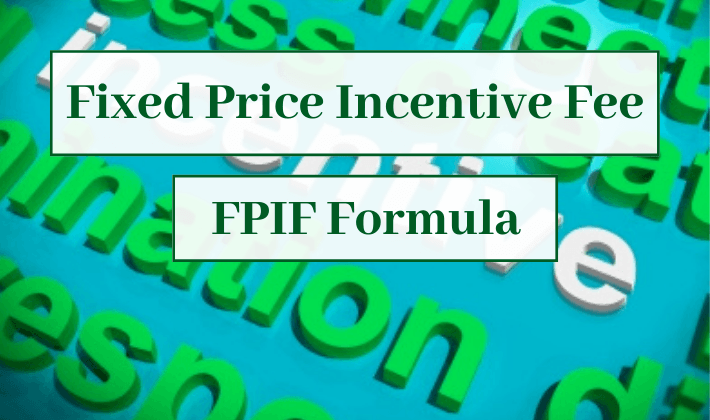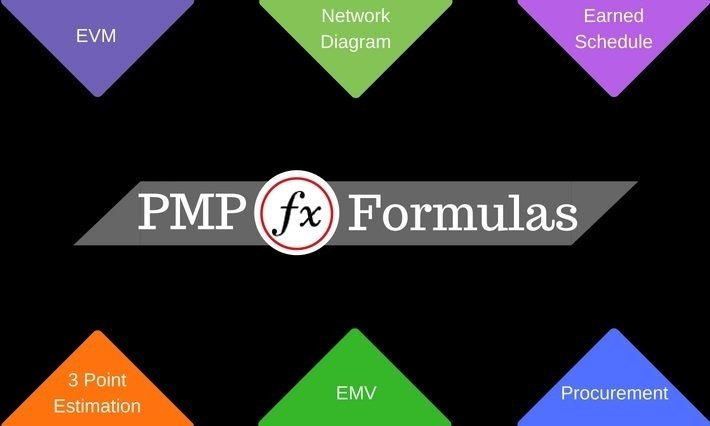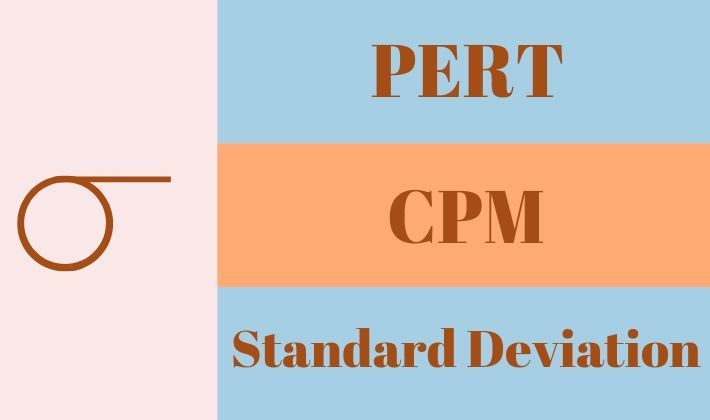## Critical Path Analysis Example – 2 Ways to Calculate Critical Path

Critical Path Analysis Example – Using 0 and 1 Method I got introduced to Critical Path Analysis in the year 1997. I am a software engineer by education. I learned most of the project management concepts while managing software projects – Critical Path Analysis was one such concept. I learned about Critical Path while using…## How to Remember Point of Total Assumption Formula?

Point of Total Assumption Formula in Fixed Price Incentive Fee Contract How do you remember any formula? Simple! Understand the concept you will not need to remember the formula. I heard about Point of Total Assumption when I was preparing for PMP® Exam. At the beginning, it sounded very funny. One of the meanings of…## 6 Main Formulas of a FPIF Contract

Incentive Calculation in a FPIF Contract In my previous post, I described Fixed Price Incentive Fee Contract (FPIF). In this article, I will discuss the formulas and incentive calculations for an FPIF Contract. Let me summarize the basic nature of the contract before getting into formulas and calculations. The basic nature of a FPIF Contract…## PMP Formulas Behind Contract Types

Mathematical Orientation of Contract Types Procurement Management Knowledge Area of PMBOK® Guide defines 3 different Contract Types. I have already written two articles to explain these Contract Types. Contract Types explained through an Example Contract Types for PMP® Exam I have written this article to provide a completely different perspective to the Contract Types. This…You might be looking for a PMP formulas cheat sheet that can help you to solve mathematics questions in the certification exam. In this post, you will find a comprehensive sheet having 45 project management formulas and 57 abbreviations. The sheet can be used as a quick reference pocket guide for solving calculation based numerical problems…## Earned Value Management Formulas

Quick Reference for Earned Value Management Formulas If you are looking for a comprehensive list of all the Earned Value Management Formulas, then you are at the right place. If you are preparing for PMP® Exam, you will find all the Earned Value Formulas. This article would provide you with a step up in doing…## Estimate to Complete (ETC) Formulas In Project Management

Estimate To Complete (ETC) is an estimation of funds required to complete the remaining work of a project. I have written this post to explain ETC with the help of an example. You will find a complete discourse on ETC in this post, which includes: significance of ETC in project management. definition and meaning of…## Three Point Estimate Formulas

Quick Reference on Three Point Estimate I have written a series of articles on Three Point Estimate and related concepts. Before reading this, you should look at my first article of the series. I have explained the basic concept of Three Point Estimate in my first article. I have also explained that PERT is not…## Project Effort vs Duration vs Elapsed Time With Examples

There main difference between effort, duration and elapsed time is that effort refers to the amount of exertion or work done to complete a task, duration refers to the length of (working) time to complete a task, and elapsed time is the total amount of (working and non-working) time that passes between start of an…## Using PERT & Standard Deviation For Critical Path Analysis

You would find Program Evaluation & Review Technique (PERT), Critical Path Method (CPM) and Standard Deviation in complete detail this post. IT describes how they are related to each other and how they can be used together for project schedule analysis. This article assumes that you are already know how to use PERT Formula for…